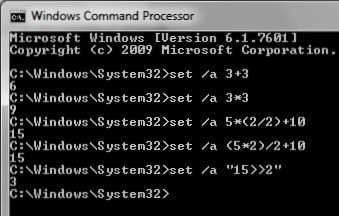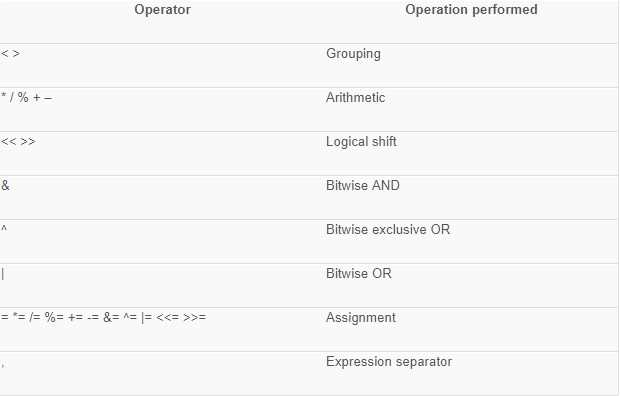# How to perform calculation ON CMD?

Updated 03-Jun-2018
Viewed 509 times

How to perform calculation ON CMD?

In general, we use a calculator or any app for doing any calculation on windows, but other than that, we can also use DOS based calculator i.e Command Prompt that works through a set of commands. Calculating arithmetic expressions on command line is more easy and expressive than other methods. Because command line comes very handy, when solving some complex logic.

The built-in DOS Shell has comprises of a mini calculator that can calculate simple arithmetic expressions on 32 bit signed integer.

The “SET” with /a switch DOS command is used to perform calculation on command prompt.

To know more on this command type set /? on the command prompt.

Below are some of the general expressions:

C:\>set /a 3+3 will output 6

C:\>set /a 3*3 will output 9

C:\>set /a 8/4 will output 2

C:\>set /a 2+2 will output 4

C:\>set /a 5*(2/2)+10 will output 15

C:\>set /a (5*2)/2+10 will output 15

C:\>set /a “15>>2” will output 3It also support other types of operators. Always remember to enclose the expression string in quotes when using logical or modulus operator.

The following table lists the operators supported for /a in descending order of precedence.Note: It overflow values for decimal integer. It removes the decimal point value and round of the integer. i.e 13/2 will result 6 instead of 6.5.